# Chemical Equilibrium Chemical equilibrium occurs when a reaction

• Slides: 14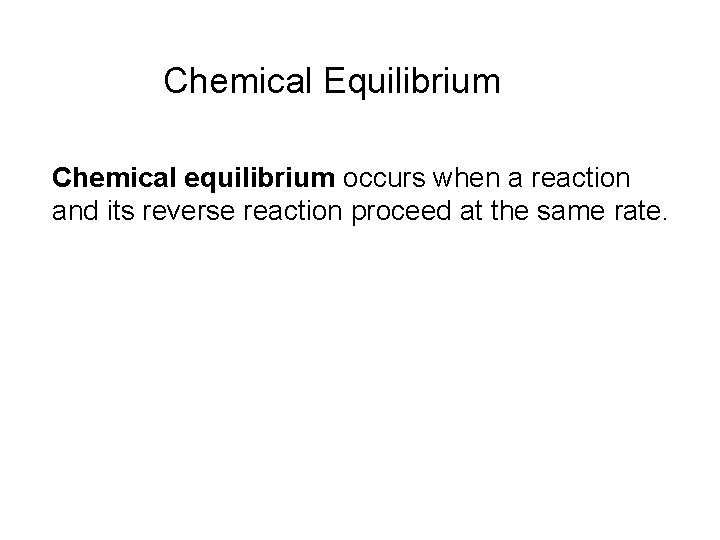Chemical Equilibrium Chemical equilibrium occurs when a reaction and its reverse reaction proceed at the same rate.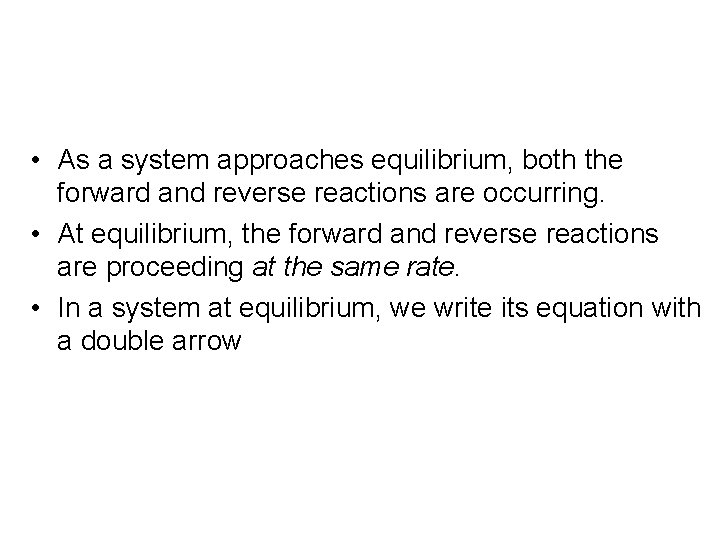• As a system approaches equilibrium, both the forward and reverse reactions are occurring. • At equilibrium, the forward and reverse reactions are proceeding at the same rate. • In a system at equilibrium, we write its equation with a double arrowØ Ø Ø At the beginning of the reaction, the rate that the reactants are changing into the products is higher than the rate that the products are changing into the reactants. When the net change of the products and reactants is zero the reaction has reached equilibrium. At equilibrium the amount of reactants and products are constant, but not necessarily equal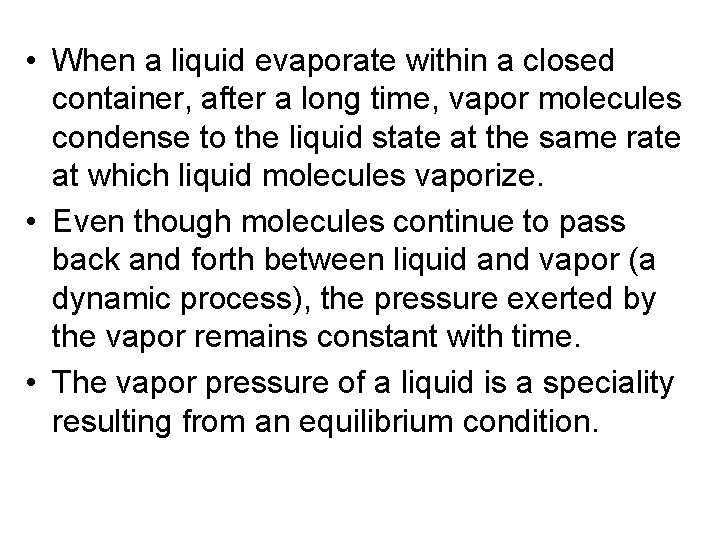• When a liquid evaporate within a closed container, after a long time, vapor molecules condense to the liquid state at the same rate at which liquid molecules vaporize. • Even though molecules continue to pass back and forth between liquid and vapor (a dynamic process), the pressure exerted by the vapor remains constant with time. • The vapor pressure of a liquid is a speciality resulting from an equilibrium condition.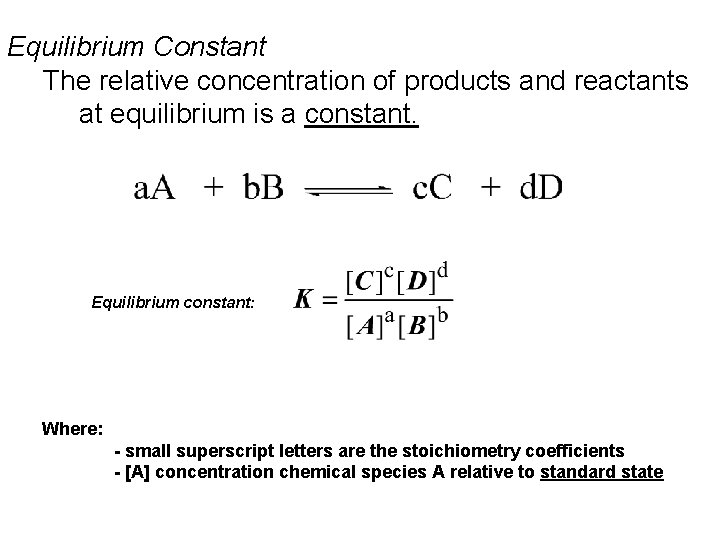Equilibrium Constant The relative concentration of products and reactants at equilibrium is a constant. Equilibrium constant: Where: - small superscript letters are the stoichiometry coefficients - [A] concentration chemical species A relative to standard stateThe Equilibrium Constant N 2 O 4(g) 2 NO 2(g) • Therefore, at equilibrium Ratef = Rater kf[N 2 O 4] = kr[NO 2]2 • Rewriting this, it becomes kf [NO 2]2 = kr [N 2 O 4]The Equilibrium Constant The ratio of the rate constants is a constant at that temperature, and the expression becomes kf [NO 2]2 Keq = = kr [N 2 O 4]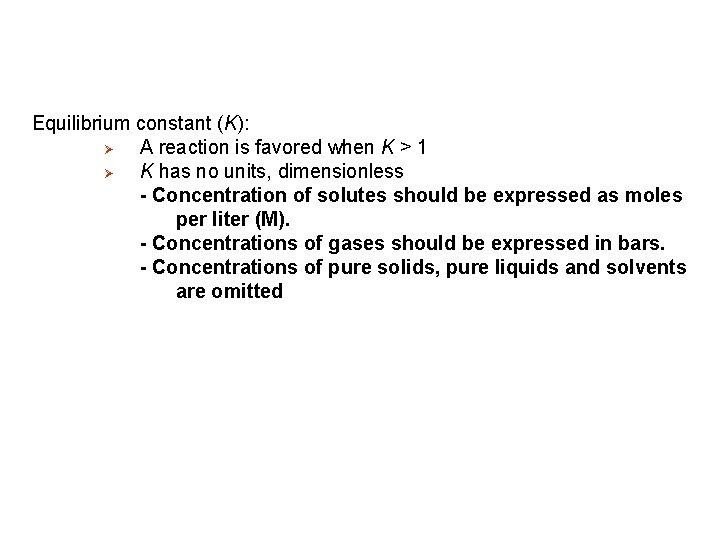Equilibrium constant (K): Ø A reaction is favored when K > 1 Ø K has no units, dimensionless - Concentration of solutes should be expressed as moles per liter (M). - Concentrations of gases should be expressed in bars. - Concentrations of pure solids, pure liquids and solvents are omitted• The expression of equilibrium constant depends on how the equilibrium equation is written. For example, for the following equilibrium: • H 2(g) + I 2(g) ⇄ 2 HI(g); • For the reverse reaction: • 2 HI(g) ⇄ H 2(g) + I 2(g); • And for the reaction: HI(g) ⇄ ½H 2(g) + ½I 2(g);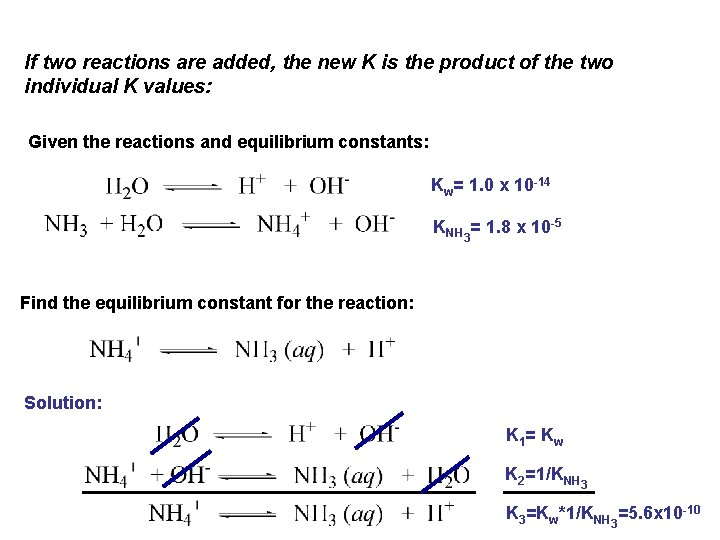If two reactions are added, the new K is the product of the two individual K values: Given the reactions and equilibrium constants: Kw= 1. 0 x 10 -14 KNH 3= 1. 8 x 10 -5 Find the equilibrium constant for the reaction: Solution: K 1 = K w K 2=1/KNH 3 K 3=Kw*1/KNH 3=5. 6 x 10 -10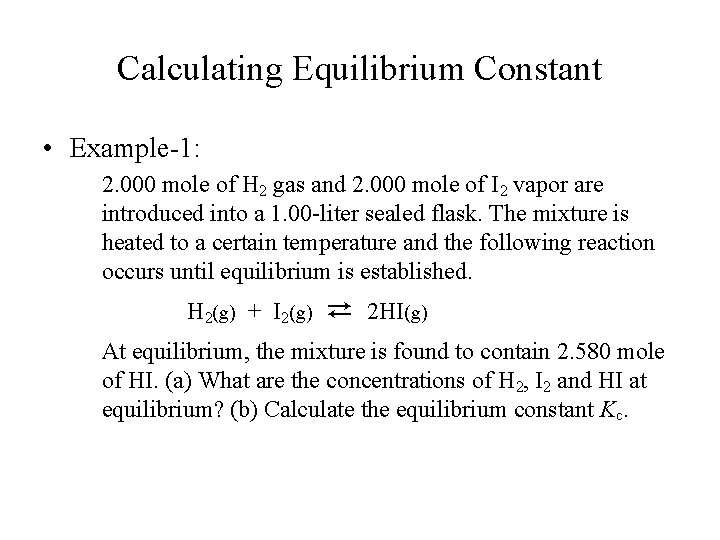Calculating Equilibrium Constant • Example-1: 2. 000 mole of H 2 gas and 2. 000 mole of I 2 vapor are introduced into a 1. 00 -liter sealed flask. The mixture is heated to a certain temperature and the following reaction occurs until equilibrium is established. H 2(g) + I 2(g) ⇄ 2 HI(g) At equilibrium, the mixture is found to contain 2. 580 mole of HI. (a) What are the concentrations of H 2, I 2 and HI at equilibrium? (b) Calculate the equilibrium constant Kc.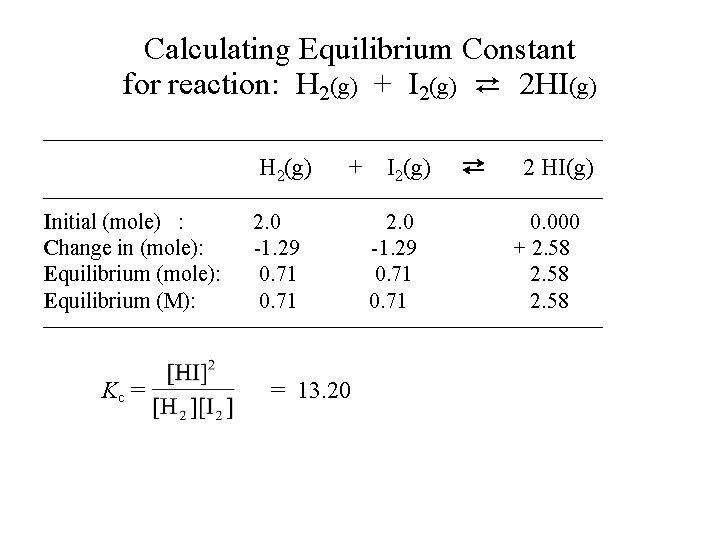Calculating Equilibrium Constant for reaction: H 2(g) + I 2(g) ⇄ 2 HI(g) —————————————— H 2(g) + I 2(g) ⇄ 2 HI(g) —————————————— Initial (mole) : Change in (mole): Equilibrium (M): 2. 0 -1. 29 0. 71 0. 000 + 2. 58 —————————————— Kc = = 13. 20Calculating Equilibrium Constant • Example-2: 1. 500 mole of HI is introduced into a 1. 00 liter sealed flask and heated to a certain temperature. Under this condition HI decomposes to produce H 2 and I 2 until an equilibrium is established. An analysis of the equilibrium mixture shows that 0. 200 mole of HI has decomposed. Calculate the equilibrium concentrations of H 2, I 2 and HI, and the equilibrium constant Kc for the following reaction: H 2(g) + I 2(g) ⇄ 2 HI(g),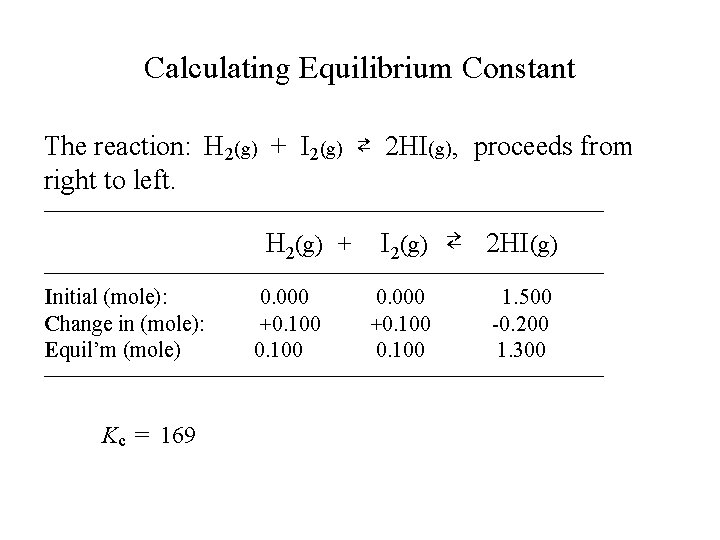Calculating Equilibrium Constant The reaction: H 2(g) + I 2(g) ⇄ 2 HI(g), proceeds from right to left. —————————————— H 2(g) + I 2(g) ⇄ 2 HI(g) —————————————— Initial (mole): Change in (mole): Equil’m (mole) 0. 000 +0. 100 1. 500 -0. 200 1. 300 —————————————— Kc = 169